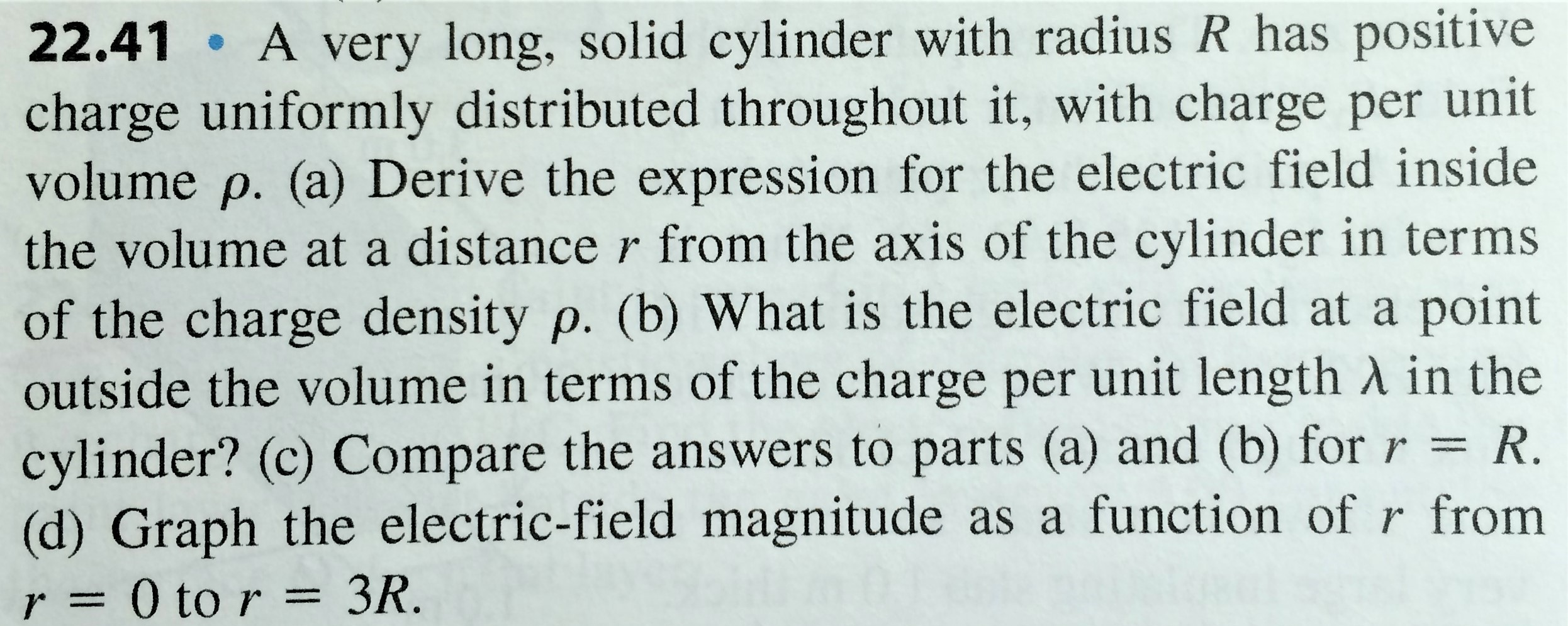# 22.41 A very long, solid cylinder with radius R has positivecharge uniformly distributed throughout it, with charge per unitvolume p. (a) Derive the expression for the electric field insidethe volume at a distance r from the axis of the cylinder in termsof the charge density p. (b) What is the electric field at a pointoutside the volume in terms of the charge per unit length A in thecylinder? (c) Compare the answers to parts (a) and (b) forr = R.(d) Graph the electric-field magnitude as a function of r from3R.r= 0 to r =

Question
408 views

(Question in image)

Hint:To check your answers to parts (a) and (b), a correct answer to part (c) should allow you to further write ρ = Q /LπR2, where Q is the total charge on the cylinder and L is the length of the cylinder.help_outlineImage Transcriptionclose22.41 A very long, solid cylinder with radius R has positive charge uniformly distributed throughout it, with charge per unit volume p. (a) Derive the expression for the electric field inside the volume at a distance r from the axis of the cylinder in terms of the charge density p. (b) What is the electric field at a point outside the volume in terms of the charge per unit length A in the cylinder? (c) Compare the answers to parts (a) and (b) forr = R. (d) Graph the electric-field magnitude as a function of r from 3R. r= 0 to r = fullscreen
check_circle

Step 1

a) Using Gauss law,

Step 2

b) Using Gauss law,

Step 3

c) When r = R,

...

### Want to see the full answer?

See Solution

#### Want to see this answer and more?

Solutions are written by subject experts who are available 24/7. Questions are typically answered within 1 hour.*

See Solution
*Response times may vary by subject and question.
Tagged in

### Electric Charges and Fields